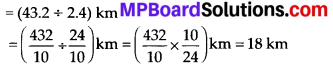## MP Board Class 7th Maths Solutions Chapter 2 Fractions and Decimals Ex 2.7

Question 1.
Find:
(i) 0.4 ÷ 2
(ii) 0.35 ÷ 5
(iii) 2.48 ÷ 4
(iv) 65.4 ÷ 6
(v) 651.2 ÷ 4
(vii) 3.96 ÷ 4
(viii) 0.80 ÷ 7
Solution: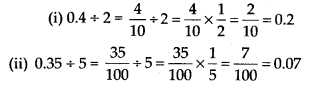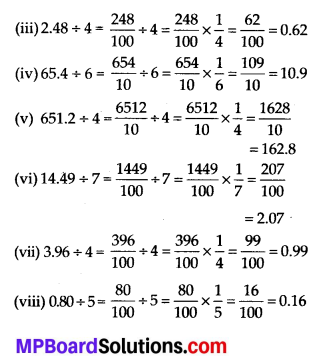Question 2.
Find:
(i) 4.8 ÷ 10
(ii) 52.5 ÷ 10
(iii) 0.7 ÷ 10
(iv) 33.1 ÷ 10
(v) 272.23 ÷ 10
(vi) 0.56 ÷ 10
(vii) 3.97 ÷ 10
Solution:
We know that when a decimal number is divided by a multiple of 10 (i.e., 10,100,1000, etc.), the decimal point will be shifted to the left by as many places as there are zeros.
(i) 4.8 ÷ 10 = 0.48
(ii) 52.5 ÷ 10 = 5.25
(iii) 0.7 ÷ 10 = 0.07
(iv) 33.1 ÷ 10 = 3.31
(v) 272.23 ÷ 10 = 27.223
(vi) 0.56 ÷ 10 = 0.056
(vii) 3.97 ÷10 = 0.397Question 3.
Find:
(i) 2.7 ÷ 100
(ii) 0.3 ÷ 100
(iii) 0.78 ÷ 100
(iv) 432.6 ÷ 100
(v) 23.6 ÷ 100
(vi) 98.53 ÷ 100
Solution:
We know that when a decimal number is divided by a multiple of 10 (i.e., 10, 100, 1000, etc.), the decimal point will be shifted to the left by as many places as there are zeros.
(i) 2.7 ÷ 100 = 0.027
(ii) 0.3 ÷ 100 = 0.003
(iii) 0.78 ÷ 100 = 0.0078
(iv) 432.6 ÷ 100 = 4.326
(v) 23.6 ÷ 100 = 0.236
(vi) 98.53 ÷ 100 = 0.9853Question 4.
Find:
(i) 7.9 ÷ 1000
(ii) 26.3 ÷ 1000
(iii) 38.53 ÷ 1000
(v) 0.5 ÷ 1000
Solution:
We know that when a decimal number is divided by a multiple of 10 (i.e., 10, 100, 1000, etc.), the decimal point will be shifted to the left by as many places as there are zeros.
(i) 7.9 ÷ 1000 = 0.0079
(ii) 26.3 ÷ 1000 = 0.0263
(iii) 38.53 ÷ 1000 = 0.03853
(iv) 128.9 ÷ 1000 = 0.1289
(v) 0.5 ÷ 1000 = 0.0005

Question 5.
Find:
(i) 7 ÷ 3.5
(ii) 36 ÷ 2
(iii) 3.25 ÷ 0.5
(iv) 30.94 ÷ 0.7
(v) 0.5 ÷ 0.25
(vi) 7.75 ÷ 0.25
(vii) 76.5 ÷ 0.15
(viii) 37.8 ÷ 1.4
(ix) 2.73 ÷ 1.3
Solution: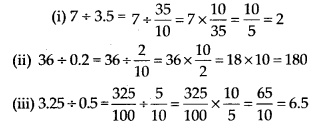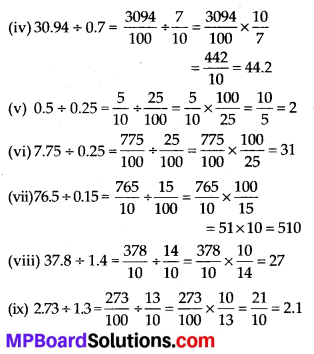Question 6.
A vehicle covers a distance of 43.2 km in 2.4 litres of petrol How much distance wilt it cover in one litre of petrol?
Solution:
Distance covered in 2.4 Litres of petrol = 43.2 km
∴ Distance covered in 1 litre of petrol = (43.2 ÷ 2.4) km